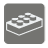[old] String Convertion
Undefined
English

You are given two strings, line1 and line2. Answer, what is the smallest number of operations you need to transform line1 to line2?

Operations are:

• Delete one letter from one of strings
• Insert one letter into one of strings
• Replace one of letters from one of strings with another letter

Input: two arguments, two strings.

Output: int, minimum number of operations.

Example:

```stepsToConvert('line1', 'line1') == 0
stepsToConvert('line1', 'line2') == 1
stepsToConvert('ine', 'line2') == 2
```

Precondition: 0 <= len(line) < 100